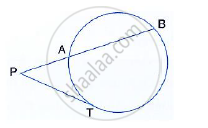Share

(Iii) in the Given Figure Tangent Pt = 12.5 Cm and Pa = 10 Cm; Find Ab. - ICSE Class 10 - Mathematics

ConceptArc and Chord Properties - If Two Chords Intersect Internally Or Externally Then the Product of the Lengths of the Segments Are Equal

Question

(iii) In the given figure tangent PT = 12.5 cm and PA = 10 cm; find AB.Solution

Since PAB is the secant and PT is the tangent
∴ PT^2 =  PA × PB
⇒ 12.5 ^2 = 10 × PB
⇒ PB (12.5 ×12.5)/10 = 15.625 cm
AB = PB - PA  = 15.625- 10 =  5.625 cm

Is there an error in this question or solution?

APPEARS IN

Solution (Iii) in the Given Figure Tangent Pt = 12.5 Cm and Pa = 10 Cm; Find Ab. Concept: Arc and Chord Properties - If Two Chords Intersect Internally Or Externally Then the Product of the Lengths of the Segments Are Equal.
S